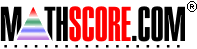Math Practice Online > free > lessons > Florida > 6th grade > Basic Distributive Property

## Basic Distributive Property

Completion of equations that demonstrate the distributive property. For example, since 2 * (3 + 4) = (2 * 3) + (2 * 4)

 Sample Problems for Basic Distributive Property Lesson for Basic Distributive Property

### This topic aligns to the following state standards

Grade 3: Num      e. applying the distributive property of multiplication, such as 8 x 7 = (8 x 5) + (8 x 2)
Grade 4: Num      c. the commutative, associative, and distributive properties of multiplication.
Grade 5: Num 5. Explains and demonstrates the commutative, associative, and distributive properties of multiplication.
Grade 6: Num 3. Knows and applies the commutative, associative, and distributive properties in the addition and multiplication of rational numbers.

Copyright Accurate Learning Systems Corporation 2008.